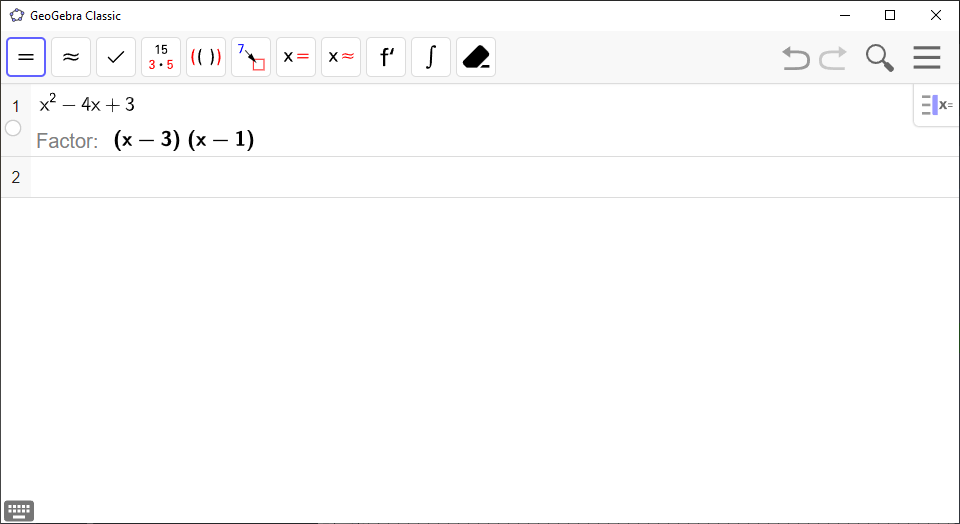Math Topics

# How to Use the CAS Calculator in GeoGebra

CAS (Computer Algebra System) is the calculator in GeoGebra, and has its own view. Open it by clicking CAS underView inMenu.

In this entry, we’ll take a look at the basics of CAS. If you want to learn how to use CAS for a specific task in a given subject, like solving systems of equations or differentiation, check out the respective GeoGebra entries. The entries are categorized by subject.

By typing your calculation into an empty row in CAS and pressing Enter, CAS will do the calculation for you.

GeoGebra Instruction 1

### Arithmetic

Open CAS underView inMenu.

• To add $36+87$, type

36 + 87

and press Enter.

• To subtract $143-46$, type

143 - 46

and press Enter.

• To multiply $13×15$, type

13 * 15

and press Enter.

• To divide $132÷12$, type

132 / 12

and press Enter.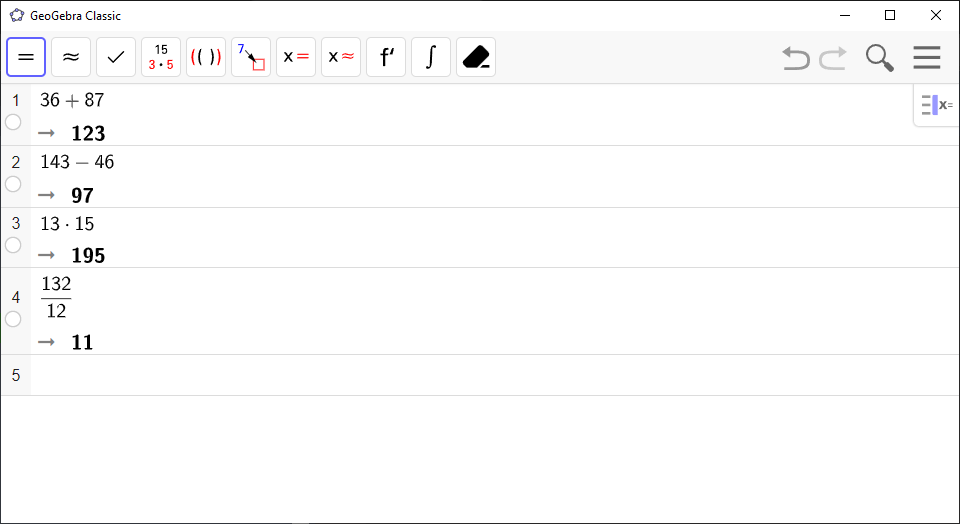GeoGebra Instruction 2

### PowersandRoots

1.
Open CAS underView inMenu.
2.
Powers:

To calculate ${x}^{p}$, type x^p and press Enter.

Roots:

To calculate ${x}^{\frac{1}{n}}$, type x^1/n and press Enter.

If want to compute a square root, you can use the command sqrt(<x>) instead. sqrt is short for “square root”.

Note! GeoGebra only prints the positive root, even if there’s a negative root too.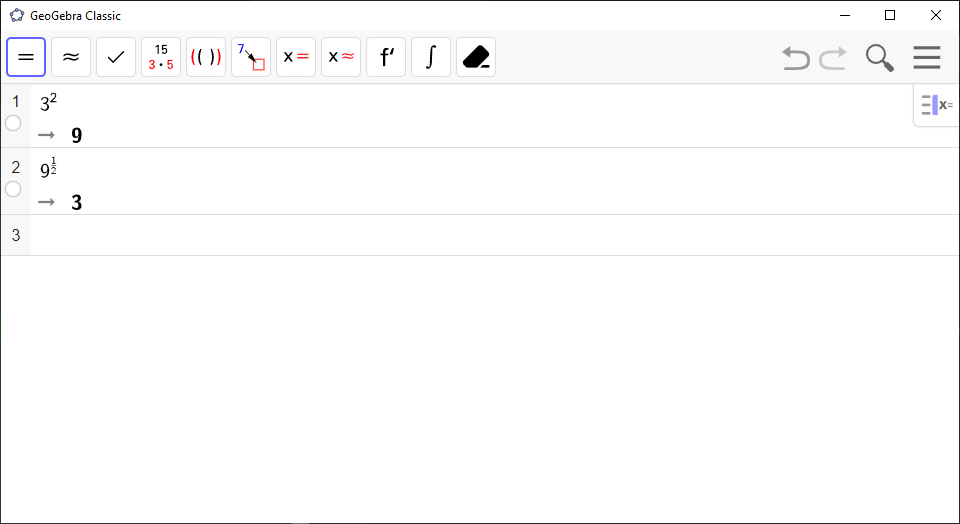Algebraic expressions work the same way, but make sure that you use the multiplication symbol (*) between the individual symbols. If you for example type ab, GeoGebra will interpret it as an object with the name “ab”, and not $a\cdot b$.

GeoGebra Instruction 3

### AlgebraicExpressions

Open CAS underView inMenu.

• To compute $2a+3a$, type

2a + 3a

and press Enter.

• To compute $ab\cdot {b}^{2}$, type

a * b * b^2

and press Enter. You get $a{b}^{3}$. If you type

ab * b^2

you get $ab\cdot {b}^{2}$, because GeoGebra interprets ab as an object, and not the product $a\cdot b$.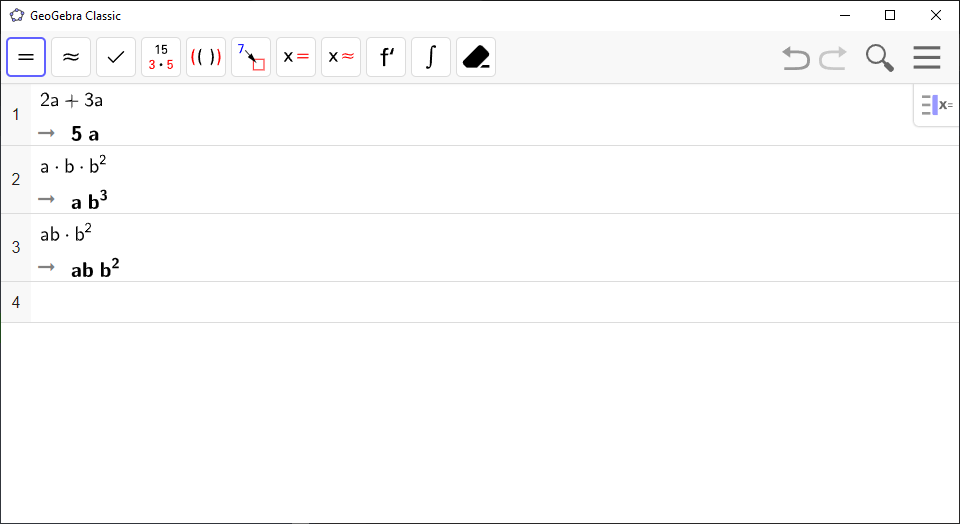If you want to type a fraction in CAS, you need to add a fraction bar (also known as “vinculum”). Here’s how you do it:

GeoGebra Instruction 4

### Fractions

1.
Open CAS underView iMenu.
2.
Type /. A fraction bar should appear, which consists of two empty fields for the numerator and the denominator, respectively. Type the numerator of your fraction first, and then click on the second field and type your denominator. Press Enter when you’re done.

Note! CAS simplifies fractions automatically.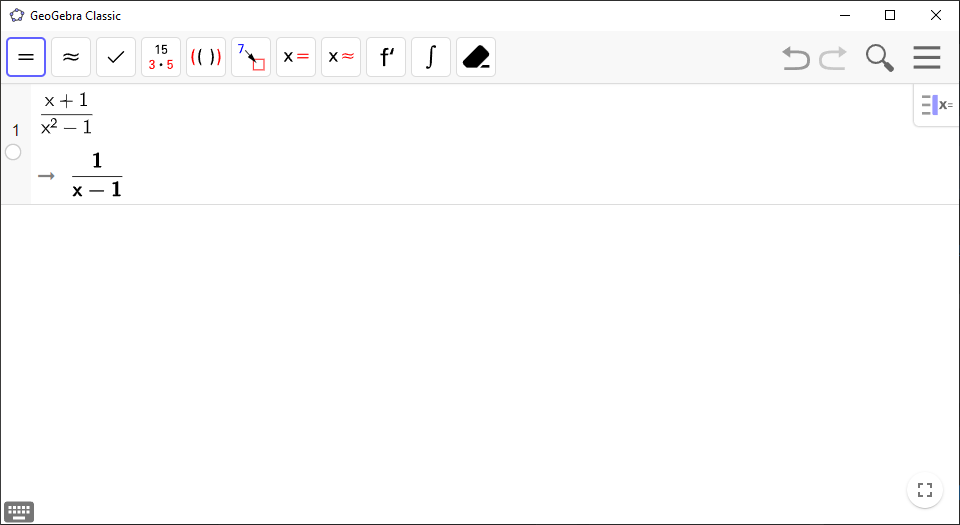CAS can also be used for rounding numbers.

GeoGebra Instruction 5

### Rounding

1.
Open CAS underView iMenu.
2.
If you want to round the number to an integer, use

round(<x>)

where you replace <x> with your number.

3.
If you want to round your number to a specific number of decimal places (lower than the number of decimal places there currently are), use

round(<x>, <y>)

where you replace <x> with your number, and <y> with the number of decimal places.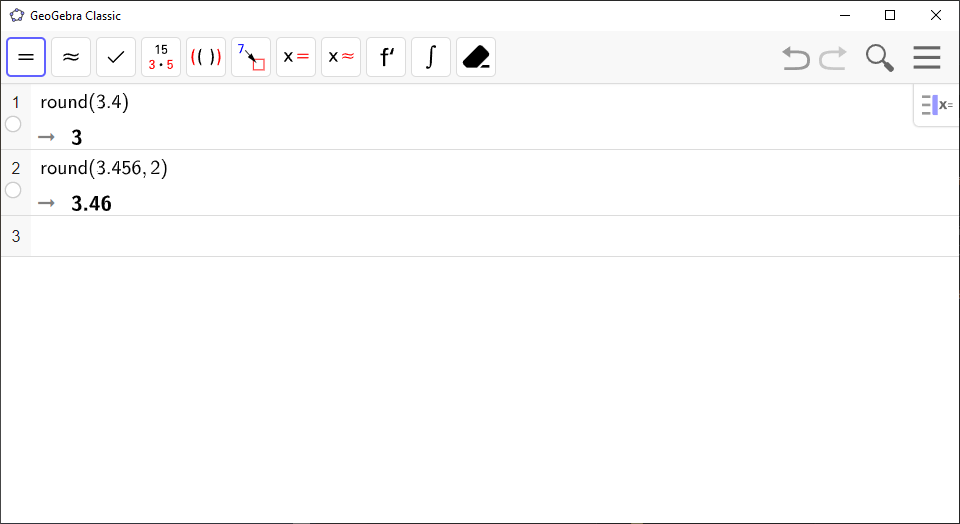You can also calculate powers and roots in CAS.

By clicking Expandin the CAS Toolbar, you can expand parentheses.

GeoGebra Instruction 6

### ExpandingParentheses

1.
Open CAS underView inMenu.
2.
Type your expression in CAS and click Expandfrom Toolbar.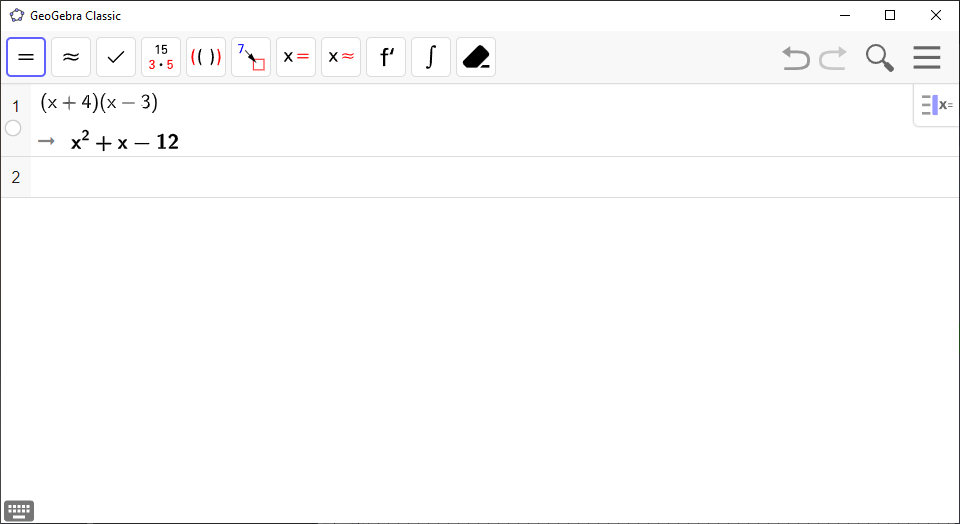CAS can be used to factorize an expression. If it doesn’t change after clicking Factor, it can’t be factorized.

Note! GeoGebra uses the term factor instead of factorize.

GeoGebra Instruction 7

### Factorization

1.
Open CAS underView inMenu.
2.
Type your expression in CAS and click Factorin Toolbar.